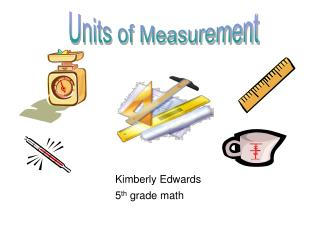DownloadDownload PresentationKimberly Edwards 5 th grade math

# Kimberly Edwards 5 th grade math

Télécharger la présentation## Kimberly Edwards 5 th grade math

- - - - - - - - - - - - - - - - - - - - - - - - - - - E N D - - - - - - - - - - - - - - - - - - - - - - - - - - -
##### Presentation Transcript

1. Units of Measurement Kimberly Edwards 5th grade math

2. Units of Measurement Length Mass Capacity Temperature Goal 2: Measurement-The learner will recognize and use standard units of metric and customary measurement. Objective 2.01: Estimate the measure of an object in one system given the measure of that object in another system.

3. Units of Length 1 centimeter (cm)=10 millimeters (mm) 1 meter (m)=100 cm or 1,000 mm 1 kilometer (km)=1,000 m 1 millimeter 1 centimeter 1 meter 1 kilometer thickness of a dime width of a height of a 6 city blocks pinky finger doorknob

4. Select millimeter, centimeter, meter, or kilometer. Soccer field A. MeterB. Kilometer

5. You are Correct!!!

6. You are Incorrect.

7. What is…. 170 mm = _____ cm A. 17 cmB. 7 cm

9. Wrong.

10. Units of Mass 1 gram (g) =1,000 milligrams (mg) 1 kilogram (kg)= 1,000 (g) 1 milligram 1 gram 1 kilogram a bread crumb a slice of bread a loaf of bread

11. Replace the space with or . 3 kg ____ 3,000 g A. B.

12. Sorry.

13. Correct!!!

14. One highlighter has a mass of 11 grams. Another highlighter has a mass of 108 milligrams. Which highlighter has the greater mass? • 11 grams B. 108 milligrams

15. Correct!!!

16. Oh No!!!

17. Units of Capacity 1 liter (L) = 1,000 milliliters (mL) 1 milliliter 1 liter amount of liquid in a medium-sized an eyedropper sports drink

18. A faucet is constantly dripping wastes about 90 liters of water every week. How many milliliters of water is this? 90 L = ___ mL • 90,000 mL B. .009 mL

19. Correct!!!

20. Sorry.

21. A container of orange juice holds 580 milliliters. How many liters is 580 milliliters? A. 0.58 L B. 5,800 L

22. Great!!!

23. Incorrect.

24. Units of Temperature Degrees are units of measurement used to describe temperature. • Celsius (˚C) • Fahrenheit (˚F)

25. Estimate each temperature in degrees Celsius. 80˚F A. 27° C B. 26.67° C

26. You’re Right!!!

27. That is Incorrect.

28. Normal body temperature for a human is 98.6°F. Lilly’s brother had a temperature of 101.3°F. How much greater is his temperature than normal body temperature? A. 2.7° F B. 3° F

29. That is Correct!!!

30. Incorrect.

31. Goodbye